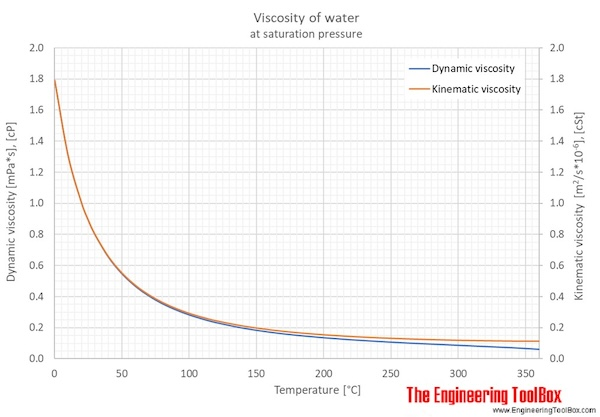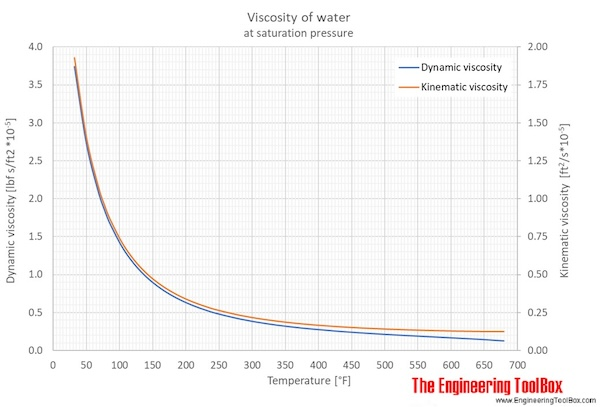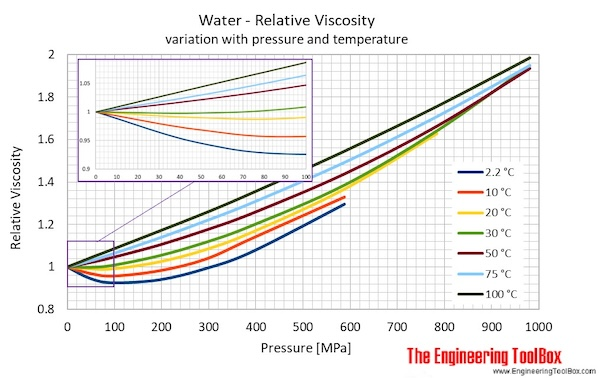Engineering ToolBox - Resources, Tools and Basic Information for Engineering and Design of Technical Applications!

# Water - Dynamic (Absolute) and Kinematic Viscosity

## Free online calculator - figures and tables with viscosity of water at temperatures ranging 0 to 360°C (32 to 675°F) - Imperial and SI Units.

The viscosity of a fluid is a measure of its resistance to gradual deformation by shear stress or tensile stress.

For further definitions, go to Absolute (dynamic) and kinematic viscosityAbsolute or dynamic viscosity is used to calculate Reynold's Number to determine if a fluid flow is laminar, transient or turbulent.

### Online Water Viscosity Calculator

The calculator below can be used to calculate the liquid water dynamic or kinematic viscosity at given temperatures.
The output dynamic viscosity is given as cP, mPa*s, Pa*s, N*s/m2,lbf*s/ft2 and lbm/(ft*h),
while the kinematic viscosity is given as cSt, m2/s, and ft2/s

Note! Temperature must be within the ranges 0-370 °C, 32-700 °F, 273-645 K and 492-1160 °R to get valid values.

Temperature

Choose the actual unit of temperature:

°C  °F  °R

See Water and Heavy Water - thermodynamic properties.

The figures and tables below shows how water viscosity changes with temperature (°C and °F) at water saturation pressure (which for practicle use, gives the same result as atmospheric pressure at temperatures < 100 °C (212°F)). One figure showing relative viscosity with variations in pressure and temperature is also included.Back to top

 Temperature Pressure Dynamic viscosity Kinematic viscosity [°C] [MPa] [Pa s], [N s/m2] [cP], [mPa s] [lbf s/ft2 *10-5] [m2/s*10-6], [cSt]) 0.01 0.000612 0.0017914 1.79140 3.7414 1.7918 10 0.0012 0.0013060 1.30600 2.7276 1.3065 20 0.0023 0.0010016 1.00160 2.0919 1.0035 25 0.0032 0.0008900 0.89004 1.8589 0.8927 30 0.0042 0.0007972 0.79722 1.6650 0.8007 40 0.0074 0.0006527 0.65272 1.3632 0.6579 50 0.0124 0.0005465 0.54650 1.1414 0.5531 60 0.0199 0.0004660 0.46602 0.9733 0.4740 70 0.0312 0.0004035 0.40353 0.8428 0.4127 80 0.0474 0.0003540 0.35404 0.7394 0.3643 90 0.0702 0.0003142 0.31417 0.6562 0.3255 100 0.101 0.0002816 0.28158 0.5881 0.2938 110 0.143 0.0002546 0.25461 0.5318 0.2677 120 0.199 0.0002320 0.23203 0.4846 0.2460 140 0.362 0.0001966 0.19664 0.4107 0.2123 160 0.618 0.0001704 0.17043 0.3559 0.1878 180 1.00 0.0001504 0.15038 0.3141 0.1695 200 1.55 0.0001346 0.13458 0.2811 0.1556 220 2.32 0.0001218 0.12177 0.2543 0.1449 240 3.35 0.0001111 0.11106 0.2320 0.1365 260 4.69 0.0001018 0.10181 0.2126 0.1299 280 6.42 0.0000936 0.09355 0.1954 0.1247 300 8.59 0.0000859 0.08586 0.1793 0.1206 320 11.3 0.0000783 0.07831 0.1636 0.1174 340 14.6 0.0000703 0.07033 0.1469 0.1152 360 18.7 0.0000603 0.06031 0.1260 0.1143
 Temperature Pressure Dynamic viscosity Kinematic viscosity [°F] [psi] [lbf s/ft2 *10-5] [lbm/(ft h)] [cP], [mPa s] [ft2/s*10-5] 32.02 0.9506 3.7414 4.3336 1.7914 1.9287 34 0.0962 3.6047 4.1752 1.7259 1.8579 39.2 0.1180 3.2801 3.7992 1.5705 1.6906 40 0.1217 3.2340 3.7458 1.5484 1.6668 50 0.1781 2.7276 3.1593 1.3060 1.4063 60 0.2563 2.3405 2.7109 1.1206 1.2075 70 0.3634 2.0337 2.3556 0.9737 1.0503 80 0.5076 1.7888 2.0719 0.8565 0.9250 90 0.6992 1.5896 1.8411 0.7611 0.8234 100 0.9506 1.4243 1.6497 0.6820 0.7392 110 1.277 1.2847 1.4880 0.6151 0.6682 120 1.695 1.1652 1.3496 0.5579 0.6075 130 2.226 1.0620 1.2300 0.5085 0.5551 140 2.893 0.9733 1.1273 0.4660 0.5102 150 3.723 0.8950 1.0366 0.4285 0.4706 160 4.747 0.8279 0.9589 0.3964 0.4367 170 6.000 0.7698 0.8916 0.3686 0.4074 180 7.520 0.7192 0.8330 0.3444 0.3820 190 9.349 0.6745 0.7813 0.3230 0.3596 200 11.537 0.6300 0.7297 0.3016 0.3371 212 14.710 0.5881 0.6812 0.2816 0.3163 220 17.203 0.5619 0.6508 0.2690 0.3032 240 25.001 0.5050 0.5850 0.2418 0.2750 260 35.263 0.4575 0.5299 0.2191 0.2515 280 49.286 0.4176 0.4837 0.2000 0.2320 300 67.264 0.3840 0.4448 0.1839 0.2157 350 134.73 0.3202 0.3708 0.1533 0.1853 400 247.01 0.2750 0.3185 0.1317 0.1648 450 422.32 0.2404 0.2785 0.1151 0.1504 500 680.56 0.2126 0.2463 0.1018 0.1398 550 1045.0 0.1888 0.2187 0.0904 0.1322 600 1542.1 0.1673 0.1937 0.0801 0.1270 625 1851.2 0.1562 0.1809 0.0748 0.1252 650 2207.8 0.1438 0.1666 0.0689 0.1239 675 2618.7 0.1292 0.1496 0.0619 0.1230

### Unit conversion

Viscosity Dynamic, Absolute
centipoise [cP], gram/(centimetre second) [g/(cm s)] = poise [P],  kilogram/metre second [kg/m s]=newton second/square metre [N s/m2]= pascal second [Pa s], pound/(foot hour) [lb/(ft h)], pound/(foot second) [lb/(ft s)], reyn [reyn]

• 1 cP = 0.001 Pa s = 0.01 P = = 0.01 g/(cm sec) = 6.72197x10-4 lb/(ft s) = 2.4191 lb/(ft h)
• 1 lb/(ft h) = 0.00027778 lb/(ft s) = 0.00041338 Pa s = 0.0041338 P = 0.41338 cP
• 1 lb/(ft s) = 3600 lb/(ft h) = 1.48816 Pa s = 14.8816 P = 1488.16 cP
• 1 kg/(m s ) = 1 (N s)/m2 = 1 Pa s = 10 P = 1000 cP = 0.672197 lb/(ft s) = 2419.09 lb/(ft h)
• 1 (N s)/m2 = 1 kg/(m s ) = 1 Pa s = 10 P = 1000 cP = 0.672197 lb/(ft s) = 2419.09 lb/(ft h)
• 1 P = 1 g/(cm s) = 0.1 Pa s = 100 cP = 0.067197 lb/(ft s) = 241.909 lb/(ft h)
• 1 Pa s = 1 kg/(m s) = 1 (N s)/m2 = 10 P = 1000 cP = 0.672197 lb/(ft s) = 2419.08 lb/(ft h) =  0.00014504 reyn
• 1 reyn = 6894.76 Pa s

Viscosity Kinematic
centistoke [cSt] = square millimetre/second [mm2/s], square foot/hour [ft2/h], square foot/second [ft2/s], square inch/second [in2/s], square metre/hour [m2/h], square metre/second [m2/s], stoke [St] = square centimetre/second [cm2/s]

• 1 cm2/s = 1 St = 100 mm2/s = 100 cSt = 1x10-4 m2/s = 0.36 m2/h = 1.07639x10-3 ft2/s = 3.875008 ft2/h = 0.1550003 in2/s
• 1 cSt = 1 mm2/s = 0.01 St = 1x10-6 m2/s = 0.0036 m2/h = 1.07639x10-5 ft2/s = 0.03875008 ft2/h = 0.001550003 in2/s
• 1 ft2/h = 2.7778x10-4 ft2/s = 0.04 in2/s = 2.58064x10-5 m2/s = 0.09290304 m2/h = 25.8064 cS = 0.258064 St
• 1 ft2/s = 3600 ft2/h = 144 in2/s = 0.09290304 m2/s = 334.451 m2/h = 92903.04 cSt = 929.0304 St
• 1 in2/s = 0.0069444 ft2/s = 25 ft2/h = 0.00064516 m2/s = 2.322576 m2/h = 645.16 cSt = 6.4516 St
• 1 m2/h = 1/3600 m2/s = 2.7778x10-4 m2/s = 2.7778 cm2/s = 277.78 mm2/s = 277.78 cSt = 2.7778 St = 0.00298998 ft2/s = 10.7639 ft2/h = 0.430556 in2/s
• 1 m2/s = 3600 m2/h = 1x104 cm2/s = 1x104 St = 1x106 mm2/s = 1x106 cSt = 10.7639 ft2/s = 38750.08 ft2/h = 1550003 in2/s
• 1 mm2/s = 1 cSt = 1x10-6 m2/s = 0.0036 m2/h = 0.01 cm2/s = 0.01 St = 1.07639x10-5 ft2/s = 0.03875008 ft2/h = 0.001550003 in2/s
• 1 St = 1 cm2/s = 100 cSt = 100 mm2/s = 1x10-4 m2/s = 0.36 m2/h = 1.076x10-3 ft2/s = 3.875008 ft2/h = 0.1550003 in2/s

### Related Mobile Apps from The Engineering ToolBox- free apps for offline use on mobile devices.

## Related Topics

• Fluid Mechanics - The study of fluids - liquids and gases. Involves velocity, pressure, density and temperature as functions of space and time
• Material Properties - Material properties for gases, fluids and solids - densities, specific heats, viscosities and more
• Viscosity - Documents giving viscosity of different kind of chemical species at varying conditions
• Steam and Condensate - Steam & condensate systems- properties, capacities, pipe sizing, systems configuration and more
• Water Systems - Hot and cold water service systems - design properties, capacities, sizing and more

## Engineering ToolBox - SketchUp Extension - Online 3D modeling!

Add standard and customized parametric components - like flange beams, lumbers, piping, stairs and more - to your Sketchup model with the Engineering ToolBox - SketchUp Extension - enabled for use with the amazing, fun and free SketchUp Make and SketchUp Pro .Add the Engineering ToolBox extension to your SketchUp from the SketchUp Pro Sketchup Extension Warehouse!

Translate

## Privacy

We don't collect information from our users. Only emails and answers are saved in our archive. Cookies are only used in the browser to improve user experience.

Some of our calculators and applications let you save application data to your local computer. These applications will - due to browser restrictions - send data between your browser and our server. We don't save this data.

## Citation

• Engineering ToolBox, (2004). Water - Dynamic (Absolute) and Kinematic Viscosity. [online] Available at: https://www.engineeringtoolbox.com/water-dynamic-kinematic-viscosity-d_596.html [Accessed Day Mo. Year].

Modify access date.

. .

#### Scientific Online Calculator10 15